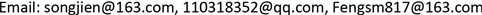1国网江苏省电力有限公司，江苏 南京

2江苏电力信息技术有限公司，江苏 南京1. 引言

2. 问题描述

3. 模型构建

1、高层货架有a排b列c层。第x排y层第z列的货位标记为(x，y，z)， x = 1 , 2 , 3 , ⋯ , a ； y = 1 , 2 , 3 , ⋯ , b ； z = 1 , 2 , 3 , ⋯ , c 。设置离出入口最近的一排为第1排，离出入口最近的一列为第1列，离地面最近的一层为第1层。

2、仓库中可存放Ｎ种不同的物料，相同种类的物料可以放在同一货位中(大小不得超过货位容量)，不允许同一货位中存放不同类型的物料。也就是一个托盘上只存放单一品种的物料。

3、每种物料的重量用M表示，物料的周转率用P表示，即某物料在一定时间内的周转次数为P。

4、每两排货架间有一巷道，巷道中有一台堆垛机。定义x方向水平运动速度是Vx；Y方向和Ｚ方向上运行速度分别为Vz和Vy

1) 出入库效率原则

f 1 = ∑ x = 1 a     ∑ y = 1 b     ∑ z = 1 c ( x v x + y v y + z v z ) ⋅ p x , y , z

2) 货架稳定性原则

f 2 = ∑ x = 1 a ∑ y = 1 b     M x , y , z ⋅ z ∑ x = 1 a ∑ y = 1 b     M x , y , z

{ min f 1 = ∑ x = 1 a     ∑ y = 1 b     ∑ z = 1 c ( x v x + y v y + z v z ) ⋅ p x , y , z min f 2 = ∑ x = 1 a ∑ y = 1 b     M x , y , z ⋅ N x , y , z ⋅ z ∑ x = 1 a ∑ y = 1 b     M x , y , z s .t .   { 1 ≤ x ≤ a 1 ≤ y ≤ b 1 ≤ z ≤ c x , y , z ∈ Z

f = ϖ 1 ∑ x = 1 a     ∑ y = 1 b     ∑ z = 1 c ( x v x + y v y + z v z ) ⋅ p x , y , z + ϖ 2 ∑ x = 1 a ∑ y = 1 b     M x , y , z ⋅ N x , y , z ⋅ z ∑ x = 1 a ∑ y = 1 b     M x , y , z

4. 算法设计

Step1：初始化t = 0，种群规模N，随机初始化精英种群 P E ( t ) ，进化种群 P M ( t ) ，搜索种群 P S ( t ) ；

Step2：计算各子种群的适应度值；

Step3：pop1锦标赛选择，pop2轮盘赌选择；

Step4：pop0互相交叉，pop1与pop1交叉得newpop1，pop2与pop2交叉得newpop2；

Step5：各种群变异；

Step6：选择前M个优秀个体产生新的精英种群 P E ( t + 1 ) ；记录 P M ( t ) 中的最优个体M-elite(t)，记录 P S ( t ) 中的最优个体S-elite(t)，并用S-elite(t)替换 P M ( t ) 中适应值最小的个体；pop1优秀个体迁移，pop2随机个体；

Step7：判断是否满足终止条件；若是，则结束；否则转到step2。

4.1. 编码设计

4.2. 归一化适应度评价函数

F = 1 ϖ 1 ∑ x = 1 a ∑ y = 1 b ∑ z = 1 c ( x v x + y v y + z v z ) ⋅ p x , y , z + ϖ 2 ∑ x = 1 a ∑ y = 1 b M x , y , z ⋅ N x , y , z ⋅ z ∑ x = 1 a ∑ y = 1 b M x , y , z + 1

4.3. 选择运算

4.4. 交叉运算

4.5. 变异运算

4.6. 移民算子

5. 实验仿真与分析

Warehouse operation parameter

Material data of upper and lower shelve

10 kV电缆中间接头，3 × 240，直通接头，冷缩，铜1836
10 kV电缆中间接头，3 × 400，直通接头，冷缩，铜30261
10 kV电缆终端，3 × 120，户内终端，冷缩，铜1010
10 kV电缆终端，3 × 400，户内终端，冷缩，铜1230
10 kV电缆终端，3 × 70，户内终端，冷缩，铜57259
10 kV电缆终端，3 × 70，设备终端，预制，铜1684
1 kV电缆终端，4 × 150，户内终端，热缩，铜5150
1 kV电缆终端，4 × 150，户外终端，冷缩，铜33
1 kV电缆终端，4 × 240，户外终端，冷缩，铜24726
1 kV电缆终端，4 × 95，户内终端，热缩，铜2040
20 kV电缆中间接头，3 × 120，直通接头，冷缩，铜1215

Continued

6. 结束语